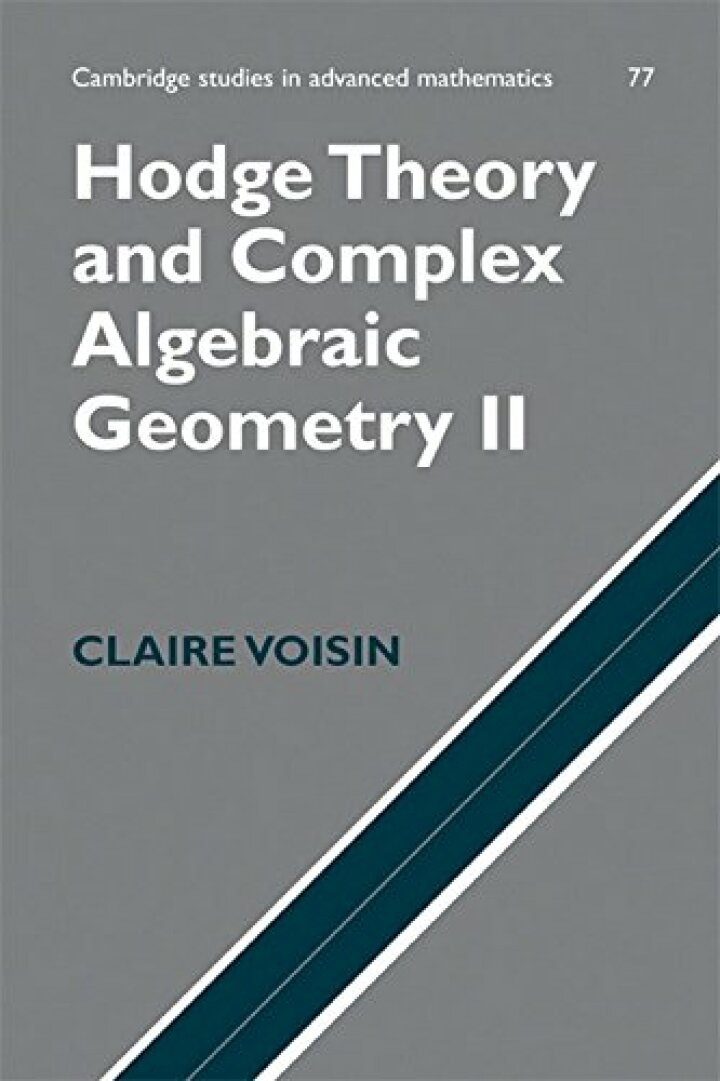# Hodge Theory and Complex Algebraic Geometry II: Volume 2

## Hodge Theory and Complex Algebraic Geometry II: Volume 2

By Claire Voisin

### Rent or Buy eTextbook

Expires on Jun 15th, 2022
\$45

Publisher List Price: \$0.00

The 2003 second volume of this account of Kaehlerian geometry and Hodge theory starts with the topology of families of algebraic varieties. Proofs of the Lefschetz theorem on hyperplane sections, the Picard–Lefschetz study of Lefschetz pencils, and Deligne theorems on the degeneration of the Leray spectral sequence and the global invariant cycles follow. The main results of the second part are the generalized Noether–Lefschetz theorems, the generic triviality of the Abel–Jacobi maps, and most importantly Nori's connectivity theorem, which generalizes the above. The last part of the book is devoted to the relationships between Hodge theory and algebraic cycles. The book concludes with the example of cycles on abelian varieties, where some results of Bloch and Beauville, for example, are expounded. The text is complemented by exercises giving useful results in complex algebraic geometry. It will be welcomed by researchers in both algebraic and differential geometry.

Subject: Mathematics & Statistics -> Post-Calculus -> Topology1st edition
Publisher: Cambridge University Press 7/3/03
Imprint: Cambridge University Press
Language: English

ISBN 10: 1139636855
ISBN 13: 9781139636858
Print ISBN: 9780521802833

Live Chats